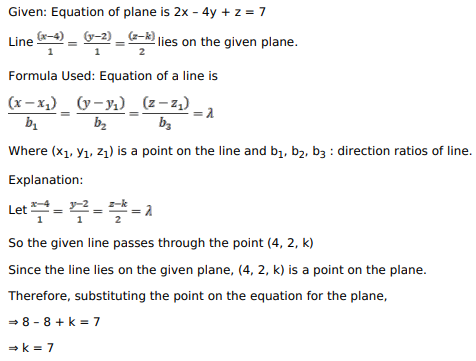# Mark against the correct answer in each of the following:

Question:

Mark against the correct answer in each of the following:

If the line $\frac{x-4}{1}=\frac{y-2}{1}=\frac{z-k}{2}$ lies in the plane $2 x-4 y+z=7$, then the value of $k$ is

A. $-7$

B. 7

C. 4

D. $-4$

Solution: